# High School Math : How to find the area of an acute / obtuse triangle

## Example Questions

### Example Question #1 : How To Find The Area Of An Acute / Obtuse Triangle

Find the area of a triangle whose base is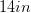and whose height is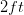.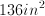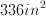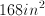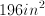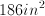Explanation:

This problem is solved using the geometric formula for the area of a triangle.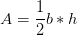Convert feet to inches.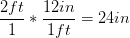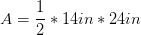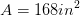### Example Question #116 : Triangles

If triangle ABC has vertices (0, 0), (6, 0), and (2, 3) in the xy-plane, what is the area of ABC?

20

12

10

18

9

9

Explanation: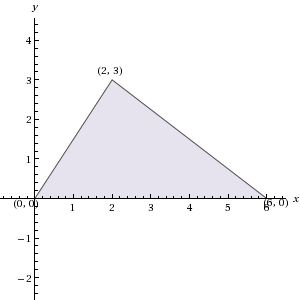Sketching ABC in the xy-plane, as pictured here, we see that it has base 6 and height 3. Since the formula for the area of a triangle is 1/2 * base * height, the area of ABC is 1/2 * 6 * 3 = 9.

### Example Question #2 : How To Find The Area Of An Acute / Obtuse Triangle

What is the area of a triangle with a height ofand a base of?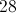Explanation:

When searching for the area of a triangle we are looking for the amount of the space enclosed by the triangle.

The equation for area of a triangle is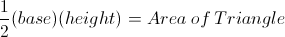Plug the values for base and height into the equation yielding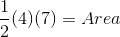Then multiply the numbers together to arrive at the answer.

### Example Question #117 : Triangles

The height,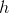, of triangle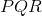in the figure is one-fourth the length of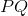. In terms of h, what is the area of triangle?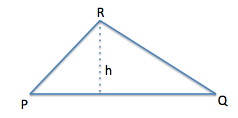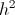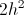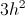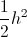If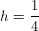*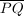, then the length ofmust be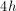.
Using the formula for the area of a triangle (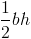), with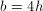, the area of the triangle must be.